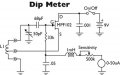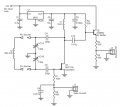# Design of Colpitts oscillator

#### EngineeringFred

Joined Sep 1, 2020
7
Hi

I've been asked to design a Colpitts oscillator for a digital grid dip meter. I do have some requirements according to frequency range and specific components. To change the frequency of the Colpitts oscillator an old air variable capacitor should be used, more specific it's a capacitor ranging from 50 pF to 500 pF. Another important detail is that the coil is meant to be changed in order to cover the frequency range. The frequency range must be at least from 35 kHz to 1 MHz.

I've have been trying for a while, but can't figure out, what I'm gonna do. I've tried designing a circuit with a JFET amplifier voltage-divider biased, but everytime I change the value of coil/inductor it all goes wrong. Is there another and better way to do it, or what am I missing/doing wrong? Appreciate any help#### Attachments

• 9.7 KB Views: 35
• 1.8 KB Views: 11

#### DickCappels

Joined Aug 21, 2008
7,973
What part of the "figuring out" is giving you trouble?

#### LvW

Joined Jun 13, 2013
1,337
I've have been trying for a while, but can't figure out, what I'm gonna do. I've tried designing a circuit with a JFET amplifier voltage-divider biased, but everytime I change the value of coil/inductor it all goes wrong. Is there another and better way to do it, or what am I missing/doing wrong? Appreciate any helpHello Fred - do you really expect any help from us ?
If yes, it is absolutely necessary to give us a MINIMUM of information.
We even do not know if your FIRST attemp (before changing the coil) was succesful.
And what means "goes wrong"?

•Delta Prime

#### EngineeringFred

Joined Sep 1, 2020
7
Sorry for leaving information out... From scratch the requirements are the following:

• Frequency range 35 kHz - 1MHz
• Various replacable coils to cover the whole frequency range
• Variable oscillator by one capacitor (50 pF to 500 pF)

From the beginning I designed a common source amplifier with a JFET. I chose to bias it by a voltage divider by using the JFET output- and transfer characteristics. I've attached those graphs as images. I would be working with a supply voltage at 9V according to a battery I have. The appropriate DC working point on the output is $$\frac{V_{CC}}{2}=4,5V$$, but to be in active/saturation region the $$V_{DS}$$ must be 4V. Because of that I chose a drain voltage at 5V, meaning $$V_D$$(voltage drop across $$R_D$$) is 4V. Therefore, the voltage drop across $$R_S$$ should be 1V.
I've determined the gate-source voltage myself to -0.6V and biased it with $$R_1$$ and $$R_2$$: $V_G=0.4V$
My question to the amplifier is, if JFET is a good solution or is a BJT better? And have I designed it correctly?

The tank circuit
I'm a little bit curious if it's the tank circuit which isn't designed correctly. I want to design the oscillator as a Colpitts where the coil is replacable. I have the air variable capacitor to varible the oscillation frequency. My question is, which capacitor (C1 or C2) is the best to use as a variable capacitor. My biggest problem is to get capacitor and inductor/coil values which results in 35 kHz, when tuning the variable capacitor to 500 pF. If I adjust the capacitor value to 50 pF I can't get oscillationens when using the same coil and C2. Right now I've used C1 as the variable capacitor. What am I missing?

Another question is why the frequency simulated isn't the same as calculated with this equation:

$f_0=\frac{1}{2\cdot \pi \cdot \sqrt{C_T\cdot L}}=\frac{1}{2\cdot \pi \cdot \sqrt{250pF\cdot 200mH}}=22.51 kHz$

Where $$C_T$$ is the total capacitance:

$C_T=\frac{C_1\cdot C_2}{C_1+C_2}=\frac{500pF\cdot 500pF}{500pF + 500pF}=250pF$

Even if I change the coil to cover a higher frequency (lower inductance) the oscillations stops in the same manner. Is there any thumb rule relation between C1 and C2?

Hope You got enough information now, if not just let me know#### EngineeringFred

Joined Sep 1, 2020
7
Sorry for leaving information out... From scratch the requirements are the following:

• Frequency range 35 kHz - 1MHz
• Various replacable coils to cover the whole frequency range
• Variable oscillator by one capacitor (50 pF to 500 pF)

From the beginning I designed a common source amplifier with a JFET. I chose to bias it by a voltage divider by using the JFET output- and transfer characteristics. I've attached those graphs as images. I would be working with a supply voltage at 9V according to a battery I have. The appropriate DC working point on the output is $$\frac{V_{CC}}{2}=4,5V$$, but to be in active/saturation region the $$V_{DS}$$ must be 4V. Because of that I chose a drain voltage at 5V, meaning $$V_D$$(voltage drop across $$R_D$$) is 4V. Therefore, the voltage drop across $$R_S$$ should be 1V.
I've determined the gate-source voltage myself to -0.6V and biased it with $$R_1$$ and $$R_2$$: $V_G=0.4V$
My question to the amplifier is, if JFET is a good solution or is a BJT better? And have I designed it correctly?

The tank circuit
I'm a little bit curious if it's the tank circuit which isn't designed correctly. I want to design the oscillator as a Colpitts where the coil is replacable. I have the air variable capacitor to varible the oscillation frequency. My question is, which capacitor (C1 or C2) is the best to use as a variable capacitor. My biggest problem is to get capacitor and inductor/coil values which results in 35 kHz, when tuning the variable capacitor to 500 pF. If I adjust the capacitor value to 50 pF I can't get oscillationens when using the same coil and C2. Right now I've used C1 as the variable capacitor. What am I missing?

Another question is why the frequency simulated isn't the same as calculated with this equation:

$f_0=\frac{1}{2\cdot \pi \cdot \sqrt{C_T\cdot L}}=\frac{1}{2\cdot \pi \cdot \sqrt{250pF\cdot 200mH}}=22.51 kHz$

Where $$C_T$$ is the total capacitance:

$C_T=\frac{C_1\cdot C_2}{C_1+C_2}=\frac{500pF\cdot 500pF}{500pF + 500pF}=250pF$

Even if I change the coil to cover a higher frequency (lower inductance) the oscillations stops in the same manner. Is there any thumb rule relation between C1 and C2?

Hope You got enough information now, if not just let me knowI forgot to mention that the frequency doesn't really change when varying C1, what can I do to make the oscillator vary more when adjusting a variable capacitor? Right now the frequency only varying 0.1 kHz per 25 pF adjusted
I really appreciate any help#### LvW

Joined Jun 13, 2013
1,337
I forgot to mention that the frequency doesn't really change when varying C1, what can I do to make the oscillator vary more when adjusting a variable capacitor? Right now the frequency only varying 0.1 kHz per 25 pF adjusted
I really appreciate any helpIs this (small) change in accordance with your theoretical calculations?

#### EngineeringFred

Joined Sep 1, 2020
7
Is this (small) change in accordance with your theoretical calculations?
Not really... Theoretically the frequency should change from 22.508 kHz (500 pF) to 23.873 kHz (400 pF), but in simulations it changes from 33.9 kHz (500 pF) to 34.3 kHz (400 pF). I've used the view FFT function in LTspice to determine the dominant frequency

#### Delta Prime

Joined Nov 15, 2019
1,188
Hello thereIs there another and better way to do it, or what am I missing/doing wrong?
Have you ever used a dip meter to
Troubleshoot maybe for antenna matching, filter trap tuning, determining unknown inductance or capacitance, measuring the length of a coaxial cable?
You get a different view when you apply and design a working schematic.
Googling dip meter circuits I found one.
It’s tuned for a range of 2 to 60 MHz so thay say.
Theoretically
I agree. Compare circuits make adjustments and submit it.
Just another way of looking at things.
P.S. when you're done I'll show you how to use it if you would like.#### BobTPH

Joined Jun 5, 2013
4,035
How are you wiring the variable capacitor wrt the two caps that form a voltage divider in a Colpitts oscillator? Across the two, or replacing one of the two?

I think it should be across the two to get the best tuning range and not disturb the feedback ratio.

Bob

#### Danko

Joined Nov 22, 2017
1,184

#### Attachments

• 1.2 KB Views: 3
• 1.4 KB Views: 1
Last edited:
•Delta Prime and SamR

#### SamR

Joined Mar 19, 2019
3,609

#### sparky 1

Joined Nov 3, 2018
611
@EngineeringFred
The original design proposed has low gain, in turn the output impedance is higher than 10k
A GDO grid dip oscillator can work on harmonics. Trying to build a GDO that was like an Eico 377 audio oscillator that is portable
uses plug-in coils and is solid state. That was quite a feat in it's day.
http://www.w6ze.org/Heathkit/Heathkit_007_HD1250.pdf
Harry Lythall - SM0VPO GDO-2 looking at the coil chart 35 Khz would mean a large coil set.
http://sm0vpo.altervista.org

Last edited:

#### EngineeringFred

Joined Sep 1, 2020
7
How are you wiring the variable capacitor wrt the two caps that form a voltage divider in a Colpitts oscillator? Across the two, or replacing one of the two?

I think it should be across the two to get the best tuning range and not disturb the feedback ratio.

Bob
I was wiring it as a replacement for one of the two. I see what you meanBy varying the capacitor C1 or C2 the feedback ratio was disturbed and might have been the issueI tried to wire the variable capacitor across both C1 and C2 (parellel) and it actually worked. I haven't thought about that... Thanks a lot

#### EngineeringFred

Joined Sep 1, 2020
7
Hello thereHave you ever used a dip meter to
Troubleshoot maybe for antenna matching, filter trap tuning, determining unknown inductance or capacitance, measuring the length of a coaxial cable?
You get a different view when you apply and design a working schematic.
Googling dip meter circuits I found one.
It’s tuned for a range of 2 to 60 MHz so thay say.
Theoretically
I agree. Compare circuits make adjustments and submit it.
Just another way of looking at things.
P.S. when you're done I'll show you how to use it if you would like.View attachment 216250

Yes, I have used one for antennas and to determine unknown inductance and capacitance. Almost every dip meter circuit I found by Google was at a frequency range above 1 MHz.
I want to design at a much lower frequency (35 kHz - 1 MHz), but I seem to get issues at this low frequency. Another thing is, the dip meter I've used was analog with a frequency dial, where this one shall be digital.Actually, I found another similar project in my search, where the dip meter is digital, but at much higher frequency. I can't figure out which components I have to adjust in order to reach the wanted frequency range without issues???#### Bordodynov

Joined May 20, 2015
2,938
It was interesting to me to develop an oscillator that meets the set conditions.
Look at what I designed:

#### Attachments

• 5.7 KB Views: 5
• 128.9 KB Views: 18

#### sparky 1

Joined Nov 3, 2018
611
Thanks a lot for every response. I ran into another problem. I've designed and simulated my circuit in LTspice where I used the MPF102 JFET. This component is in a TO-92 case and obsolete. I prefer to use a SMD component, but can't seem tro find any equivalent JFET to replace it with. Even though I find a roughly equivalent component it seems impossible to find a LTspice model to prove it works by simulation. Hope anyone can help me